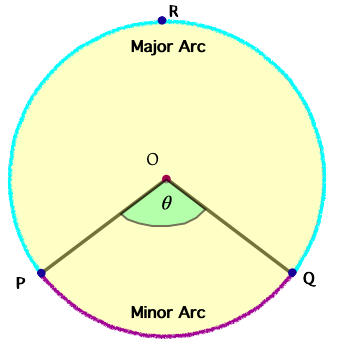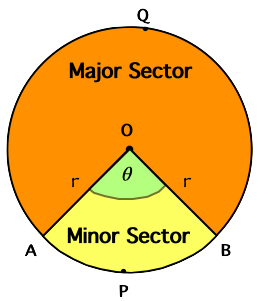# High School Geometry - Sector, Arc Length & Radian Measure

## Introduction

• Recall that a circle is a collection of all points in a plane equidistant from a fixed point.

• Degree and radian are the two units that are used to represent the measure of an angle.
• The relation between degree and the radian is summarized below.
• Angle measure in degrees $=\frac{180}{\mathrm{\pi }}×$Angle measure in radians
• Angle measure in radians $=\frac{\mathrm{\pi }}{180}×$Angle measure in degrees

## Length of Arc

• An arc is a continuous piece of a circle, as shown in the figure below.• The length of the arc (minor arc) can be found using the formula mentioned below.
•  ${L}_{arc}=\frac{\theta }{360°}×2\mathrm{\pi r}$, where $\theta$ is in degrees,
• ${L}_{arc}=r·\theta$, where $\theta$ is in radians

## Area of Sector

• The area of the Sector is the portion (part) of the circular region enclosed by two radii and the corresponding arc, as shown below.• The area of a sector (minor sector) can be found using the formula mentioned below.
•  ${A}_{sector}=\frac{\theta }{360°}×{\mathrm{\pi r}}^{2}$, where $\theta$ is in degrees,
• ${A}_{sector}=\frac{1}{2}{r}^{2}·\theta$, where $\theta$ is in radians

## Solved Examples

Example 1: Convert $\frac{\mathrm{\pi }}{9}$ radians into degree measure.

Solution:

$=20°$

Example 2: Convert $60°$ to radian measure.

Solution:

$60°=60×\frac{\mathrm{\pi }}{180}$

Example 3: A circle has a radius of 1 meter. If an arc of this circle subtends an angle $45°$ at the center O, find the length of the arc.

Solution: ${L}_{arc}=\frac{\theta }{360°}×2\mathrm{\pi r}$

$\theta =45°$

${L}_{arc}=\frac{45°}{360°}×2\mathrm{\pi }×1$

Example 4: Circle O has radius OP that measures 14 inches with a central angle $\angle POQ$, where $m\angle POQ=60°$. Find the area of the shaded sector.

Solution: ${A}_{sector}=\frac{\theta }{360°}×{\mathrm{\pi r}}^{2}$

## Cheat Sheet

• Arc length:
•  ${L}_{arc}=\frac{\theta }{360°}×2\mathrm{\pi r}$, where $\theta$ is in degrees
• ${L}_{arc}=r·\theta$, where $\theta$ is in radians
• Area of a sector:
•  ${A}_{sector}=\frac{\theta }{360°}×{\mathrm{\pi r}}^{2}$, where $\theta$ is in degrees
• ${A}_{sector}=\frac{1}{2}{r}^{2}·\theta$, where $\theta$ is in radians
• The measure of an inscribed angle is half the measure of the intercepted arc.

• The angle in a semicircle is a right angle.

## Blunder Area

• While calculating the length of the arc or area of the sector, pay attention to using the appropriate formulas depending on the unit of measure of the angle.
• When the diameter of a circle is given, sometimes students put diameter in place of radius in the formulas.